# For or While loop to print Numbers from 1 to 10 in PythonLast updated: Feb 19, 2023
6 min## #Using a For loop to print the numbers from 1 to 10

Use the `range()` class to loop from 1 to 10 in a `for` loop, e.g. `for num in range(1, 11):`.

The `range` class takes `start` (inclusive) and `stop` (exclusive) arguments and enables us to loop a specific number of times in `for` loops.

main.py
```Copied!```# ✅ for loop 1 to 10 (including 10)

for num in range(1, 11):
print(num)

# 👇️ [1, 2, 3, 4, 5, 6, 7, 8, 9, 10]
print(list(range(1, 11)))
``````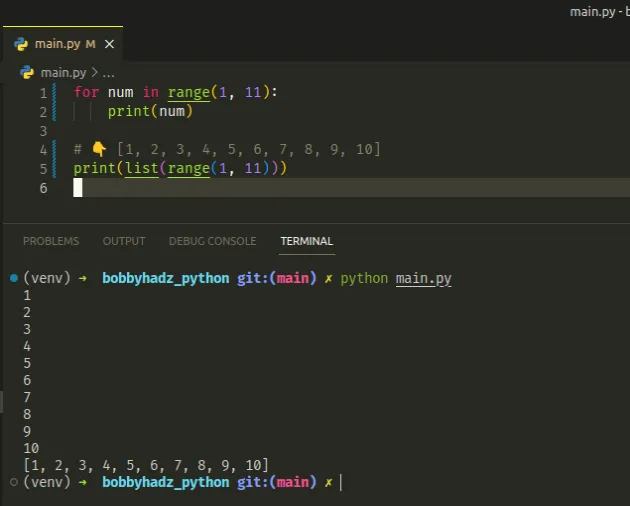If you need to exclude 10 from the range, use the following code sample instead.

main.py
```Copied!```# ✅ for loop 1 to 10 (excluding 10)

for num in range(1, 10):
print(num)

# 👇️ [1, 2, 3, 4, 5, 6, 7, 8, 9]
print(list(range(1, 10)))
``````

We used the `range()` class to loop from `1` to `10` in a for loop.

Note that the first parameter of the `range()` class (the `start` value) is inclusive, whereas the `stop` value is exclusive.

The range class is commonly used for looping a specific number of times in `for` loops and takes the following arguments:

NameDescription
`start`An integer representing the start of the range (defaults to `0`)
`stop`Go up to, but not including the provided integer
`step`Range will consist of every N numbers from `start` to `stop` (defaults to `1`)

If you only pass a single argument to the `range()` constructor, it is considered to be the value for the `stop` parameter.

main.py
```Copied!```for num in range(10):
print(num)

# 👇️ [0, 1, 2, 3, 4, 5, 6, 7, 8, 9]
print(list(range(10)))
``````
The example shows that if the `start` argument is omitted, it defaults to `0` and if the `step` argument is omitted, it defaults to `1`.

Note that the `range()` class returns a `range` object, not a list.

main.py
```Copied!```# 👇️ range(0, 10)
print(range(10))

# 👇️ [0, 1, 2, 3, 4, 5, 6, 7, 8, 9]
print(list(range(10)))
``````

If you need to convert the `range` object to a list, pass it to the list() class.

## #The `stop` value is exclusive (up to, but not including)

If values for the `start` and `stop` parameters are provided, the `start` value is inclusive, whereas the `stop` value is exclusive.

main.py
```Copied!```# 👇️ for loop 1 to 10 (including 10)
for num in range(1, 11):
print(num)

# 👇️ [1, 2, 3, 4, 5, 6, 7, 8, 9, 10]
print(list(range(1, 11)))
``````

If you need to exclude `10` from the range that is being iterated, use a `stop` value of `10`.

main.py
```Copied!```for num in range(1, 10):
print(num)

# 👇️ [1, 2, 3, 4, 5, 6, 7, 8, 9]
print(list(range(1, 10)))
``````

## #Using a While loop to print the numbers from 1 to 10

To print the numbers from 1 to 10 in a `while` loop:

1. Declare a new variable and initialize it to 1.
2. Use a while loop to iterate for as long as the variable is less than or equal to `10`.
3. Increment the variable by 1 on each iteration.
main.py
```Copied!```number = 1

while number <= 10:
print(number)
number += 1
``````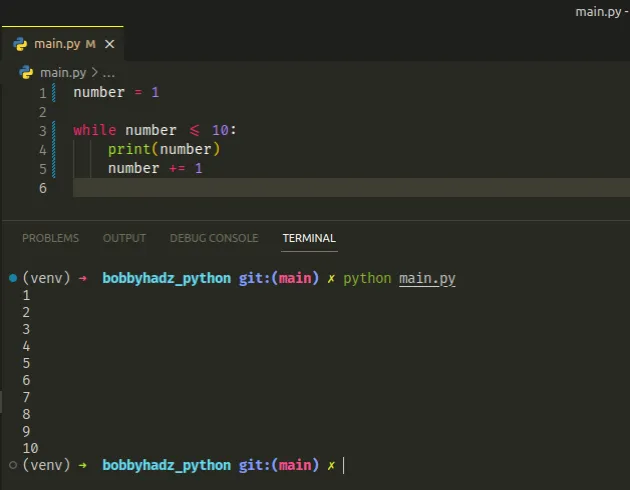We declared a new variable and initialized it to 1.

The `while` loop iterates for as long as the `number` variable is less than or equal to `10`.

On each iteration, we print the current value and increment the variable by 1.

Once the `number` variable is equal to `11`, the condition is no longer met and we exit the `while` loop.

## #Using a while True loop to print the numbers from 1 to 10

You can also use a `while True` loop to print the numbers from 1 to 10 in Python.

main.py
```Copied!```number = 1

while True:
if number > 10:
break

print(number)
number += 1
``````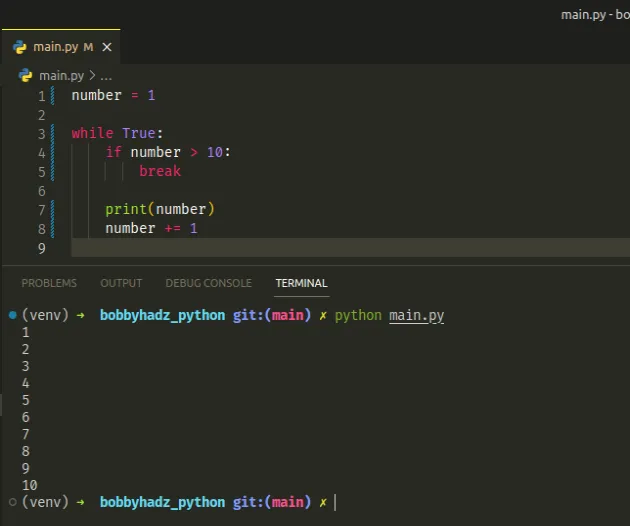The `while True` loop iterates until the `break` statement is used.

We initialized the `number` variable to 1 just like we did in the previous example.

On each iteration of the `while` loop, we check if the number variable is greater than 10.

If the condition is met, we use the `break` statement to exit the `while` loop.

The break statement breaks out of the innermost enclosing `for` or `while` loop.

If the condition isn't met, the `number` variable is in the specified range (1 to 10), so we print its value and increment it by 1.

## #Using a For loop to print the numbers from 10 to 1

To print the numbers from 10 to 1 using a `for` loop:

1. Use the `range` class to get a range of the numbers from 1 to 10.
2. Use the `reversed()` function to reverse the range.
3. Use a `for` loop to iterate over the range from 10 to 1.
main.py
```Copied!```for num in reversed(range(1, 11)):
print(num)

# 👇️ [10, 9, 8, 7, 6, 5, 4, 3, 2, 1]
print(list(reversed(range(1, 11))))
``````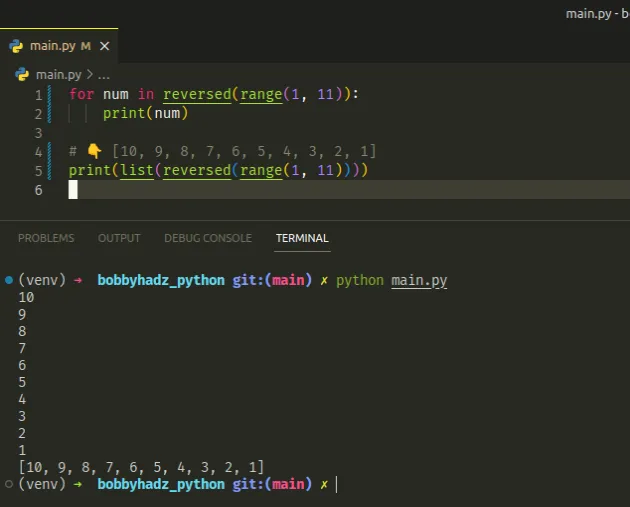We used the `range` class to get a `range` object containing the numbers from `1` to `10`.

main.py
```Copied!```# 👇️ [1, 2, 3, 4, 5, 6, 7, 8, 9, 10]
print(list(range(1, 11)))
``````

The next step is to use the `reversed()` function to reverse the range.

main.py
```Copied!```# 👇️ [10, 9, 8, 7, 6, 5, 4, 3, 2, 1]
print(list(reversed(range(1, 11))))
``````

The reversed function takes an iterator, reverses it and returns the result.

Once the `range` is reversed, we can use a `for` loop to iterate and print the numbers from `10` to `1`.

## #Using a While loop to print the numbers from 10 to 1

To print the numbers from 10 to 1 using a `while` loop:

1. Declare a new variable and initialize it to 10.
2. Use a `while` loop to iterate for as long as the variable's value is greater than or equal to `1`.
3. Print the value of the variable and decrement it by `1`.
main.py
```Copied!```number = 10

while number >= 1:
print(number)

number -= 1
``````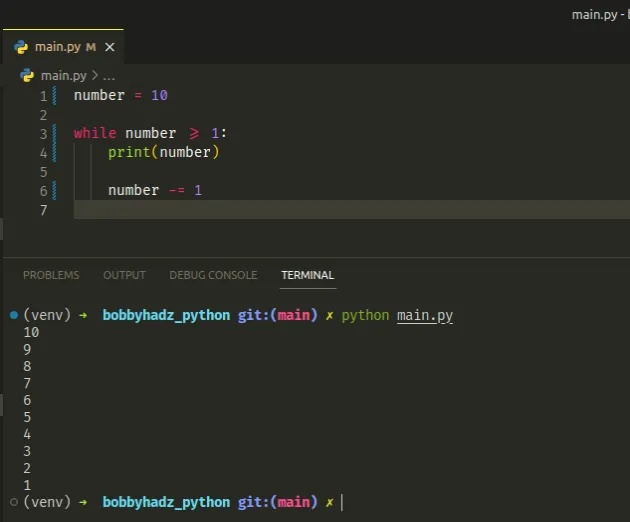The `number` variable is initially set to `10`.

On each iteration of the `while` loop, we print the current value of the variable and decrement it by `1`.

Once the `number` variable is set to `0`, the condition is no longer met and we exit the `while` loop.

## #Using a While True loop to print the numbers from 10 to 1

You can also use a `while True` loop to print the numbers from `10` to `1`.

main.py
```Copied!```number = 10

while True:
if number < 1:
break
print(number)

number -= 1
``````

The `while True` loop iterates until the `break` statement is used.

On each iteration, we check if the `number` variable is less than `1`.

If the condition is met, we use the `break` statement to exit the loop.

Otherwise, we print the variable and decrement its value by `1`.

Once the `number` variable is set to `0`, the condition is no longer met and we exit the `while` loop.